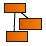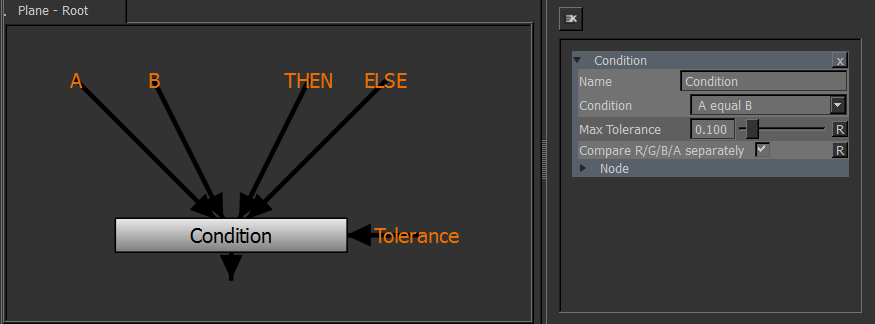﻿ Math Nodes

# Math Nodes

 Math Nodes by Jens Kafitz.

• Where to find it: (These Nodes are available in the Nodegraph only)

•NodeGraph / Right Mouse Click / Add Nodes / Math / Extension Pack /

Math Nodes allow for limited 'visual coding' in the Mari Nodegraph giving you access to more functionality and more

advanced graph generation. A variety of Nodes were added as part of MARI Extension Pack:

 Condition

The Condition Node is one of the most useful of the new Math nodes, allowing you to create an IF Statement

via the Nodegraph.It allows for the following conditions by changing the dropdown in the node:

• A equal to B
• A not equal to B
• A greater than B
• A smaller than B

A Max Tolerance Option allows you to set a range around each value that is considered acceptable

making the IF Statement true.

The Compare R/G/B/A separately option allows you to specify at what level the Inputs (A/B) are evaluated.

• With Compare R/G/B/A separately ON each channel on A will be compared to the corresponding Channel on B

When the Condition is not met the Channel will be switched to ELSE.

Subsequently this can mean that a RED Channel is switched to ELSE, while a BLUE Channel is kept at THEN.

• With Compare R/G/B/A separately OFF the condition is only met when all Channels (R,G,B and A) meet

the criterion. The result of the Node will either be fully THEN or fully ELSE.

 Log

log returns the natural logarithm of x, i.e. the value y which satisfies x=ey. The result is undefined if x≤0

 Log2

log2 returns the base 2 logarithm of x, i.e. the value y which satisfies x=2y. The result is undefined if x≤0.

 Exp

exp returns the natural exponentiation of x. i.e., ex.

 Exp2

exp2 returns 2 raised to the power of x. i.e., 2x.

 Pow

pow returns the value of x raised to the y power, i.e. xy. The result is undefined if x<0 or if x=0 and y≤0.

 DotProduct

dot returns the dot product of two vectors, x and y. i.e., x.y+x.y+...

 Length

length returns the length of the vector, i.e. sqrt { { x }^2 + { x }^2 + \dots }

 Distance

distance returns the distance between the two points point1 and point2. i.e., length(point1, point2);

 Sqrt

sqrt returns the square root of x, i.e. the value x√. The result is undefined is x<0.

 InverseSqrt

inversesqrt returns the inverse of the square root of x; i.e. the value 1 over { \sqrt x }. The result is undefined if x≤0.

Created with the Personal Edition of HelpNDoc: Free EPub and documentation generator